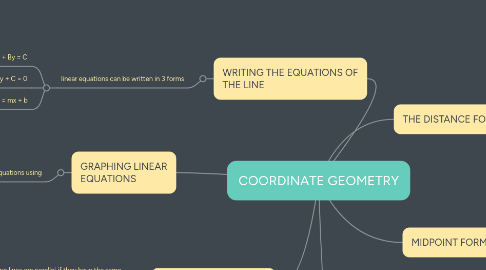# COORDINATE GEOMETRYSanjay V
Get Started. It's FreeCOORDINATE GEOMETRY by## 3. WRITING THE EQUATIONS OF THE LINE

### 3.1. linear equations can be written in 3 forms

3.1.1. Standard Form : Ax + By = C

3.1.2. General Form : Ax + By + C = 0

3.1.3. Slope - Intercept Form : y = mx + b

## 4. GRAPHING LINEAR EQUATIONS

### 4.1. we can graph linear equations using

4.1.1. x - and y intercepts

4.1.2. a point and slope of the line

4.1.3. two points of the line

## 5. PARALLEL AND PERPENDICULAR LINES

### 5.1. Two lines are parallel if they have the same slope and different y - intercepts

5.1.1. slope of L1 = slope L2

### 5.2. Two lines are perpendicular if their slopes are opposite reciprocals

5.2.1. the product of their slopes is always equal to -1